##### Member
VIP
••Mr_Wheeler, Adeodatus, Mortimer and 2 others
@pk1729
Code:
``````# # Sources:
# best with 5 mins timeframe.
# coded by TWOO
# https://www.spglobal.com/spdji/en/indices/equity/sp-500/#data
# sector-breakdown

declare lower;
input open_time = 930;

input xlpwt = 6.2; #ConsumerStaples
input xluwt = 2.6; #Utilities
input xlcwt = 9.6; #Communications
input xlfwt = 11.5; #Financials
input xlbwt = 2.6; #Materials
input xliwt = 8.0; #Industrials
input xlewt = 3.7; #Energy
input xlrewt = 2.6; #RealEstate
input xlvwt = 13.3; #HealthCare
input xlkwt = 28.1; #InformationTechnology
input xlywt = 11.8; #ConsumerDiscretionary

def SectorCount = 11;
def Scale = 5000;

#SP500 ETF sectors percent change
script PC {
input Symbol = "SPX";
def isFirstBar = GetTime() == RegularTradingStart(GetYYYYMMDD()) + 1;
def O = if isFirstBar then close(Symbol) else O;
def C = close(Symbol);
plot PctChg = (C - O) / O;
}
def xlkPctChg = PC("XLK");
def xlyPctChg = PC("XLY");
def xlvPctChg = PC("XLV");
def xlfPctChg = PC("XLF");
def xlcPctChg = PC("XLC");
def xliPctChg = PC("XLI");
def xlpPctChg = PC("XLP");
def xlrePctChg = PC("XLRE");
def xlePctChg = PC("XLE");
def xlbPctChg = PC("XLB");
def xluPctChg = PC("XLU");

def xlkSizing = xlkPctChg * xlkwt;
def xlvSizing = xlvPctChg * xlvwt;
def xlySizing = xlyPctChg * xlywt;
def xlfSizing = xlfPctChg * xlfwt;
def xlcSizing = xlcPctChg * xlcwt;
def xliSizing = xliPctChg * xliwt;
def xlpSizing = xlpPctChg * xlpwt;
def xleSizing = xlePctChg * xlewt;
def xlreSizing = xlrePctChg * xlrewt;
def xlbSizing = xlbPctChg * xlbwt;
def xluSizing = xluPctChg * xluwt;
def combinedSizing = Scale * (
xlkSizing +
xlvSizing +
xlySizing +
xlfSizing +
xlcSizing +
xliSizing +
xlpSizing +
xleSizing +
xlreSizing +
xlbSizing +
xluSizing
) / SectorCount;

plot Weighted_AD = if !IsNaN(combinedSizing) then combinedSizing else Double.NaN;

#Zeroline
plot zero = 0;
zero.SetDefaultColor(Color.GRAY);
zero.HideBubble();
zero.HideTitle();

def spxcombinedSizing = Scale *
(xlkPctChg +
xlvPctChg +
xlyPctChg +
xlfPctChg +
xlcPctChg +
xliPctChg +
xlpPctChg +
xlePctChg +
xlrePctChg +
xlbPctChg +
xluPctChg);

def NDXPctChg = PC("NDX") * 35000;
plot Non_Weighted_NDX = NDXPctChg   ;
Non_Weighted_NDX.SetDefaultColor(Color.CYAN);
Non_Weighted_NDX.SetLineWeight(1);
Non_Weighted_NDX.Hide();
Non_Weighted_NDX.HideBubble();
Non_Weighted_NDX.HideTitle();

AddVerticalLine(( GetDay() <> GetDay()), "", Color.YeLLOW, Curve.SHORT_DASH);

#SP500 ETF sectors BUBBLE
Script pct {
input Symbol = "SPX";
def aggregationPeriod = AggregationPeriod.DAY;
def price = open(Symbol, period = aggregationPeriod);
def diff = close (Symbol) - open(Symbol, period = aggregationPeriod);
plot d_pct = 100 * diff / price;
}

def XLK_d_pct = pct(“XLK”)  ;
def XLV_d_pct = pct(“XLV”)  ;
def XLY_d_pct = pct(“XLY”)  ;
def XLC_d_pct = pct(“XLC”)  ;
def XLF_d_pct = pct(“XLF”)  ;
def XLI_d_pct = pct(“XLI”)  ;
def XLP_d_pct = pct(“XLP”)  ;
def XLRE_d_pct = pct(“XLRE”)  ;
def XLB_d_pct = pct(“XLB”)  ;
def XLU_d_pct = pct(“XLU”)  ;
def XLE_d_pct = pct(“XLE”)  ;

AddLabel(yes, "InfoTech 29.2%: " + XLK_d_pct,  (if xlk_d_pct > 0 then Color.GREEN else if xlk_d_pct < 0 then color.RED else Color.gray));

AddLabel(yes, "Healthcare 13.3%: " + XLV_d_pct, (if xlv_d_pct > 0 then Color.GREEN else if xlv_d_pct < 0 then color.RED else Color.gray));

AddLabel(yes, "ConsDisc 12.5%: " + XLY_d_pct, (if xly_d_pct > 0 then Color.GREEN else if xly_d_pct < 0 then color.RED else Color.gray));

AddLabel(yes, "Comms 10.2%: " + XLC_d_pct, (if xlc_d_pct > 0 then Color.GREEN else if xlc_d_pct < 0 then color.RED else Color.gray));

AddLabel(yes, "Financials 10.7%: " + XLF_d_pct, (if xlf_d_pct > 0 then Color.GREEN else if xlf_d_pct < 0 then color.RED else Color.gray));

AddLabel(yes, "Industrials 7.8%: " + XLI_d_pct,(if xli_d_pct > 0 then Color.GREEN else if xli_d_pct < 0 then color.RED else Color.gray));

AddLabel(yes, "ConsStaples 5.9%: " + XLP_d_pct, (if xlp_d_pct > 0 then Color.GREEN else if xlp_d_pct < 0 then color.RED else Color.gray));

AddLabel(yes, "RealEstate 2.8%: " + XLRE_d_pct,(if xlre_d_pct > 0 then Color.GREEN else if xlre_d_pct < 0 then color.RED else Color.gray));

AddLabel(yes, "Materials 2.6%: " + XLB_d_pct, (if xlb_d_pct > 0 then Color.GREEN else if xlb_d_pct < 0 then color.RED else Color.gray));

AddLabel(yes, "Utilities 2.5%: " + XLU_d_pct, (if xlu_d_pct > 0 then Color.GREEN else if xlu_d_pct < 0 then color.RED else Color.gray));

AddLabel(yes, "Energy 2.7%: " + XLE_d_pct,(if xle_d_pct > 0 then Color.GREEN else if xle_d_pct < 0 then color.RED else Color.gray));plot Data = close``````

Last edited by a moderator:
Looking for the same thing. I'm both impressed, and confused, as to how they were able to specify the ADSPD for particular sectors, instead of the broader market. Any tips or clues would be appreciated.

•JohnDev1
Looking for the same thing. I'm both impressed, and confused, as to how they were able to specify the ADSPD for particular sectors, instead of the broader market. Any tips or clues would be appreciated.
They use these 11 sectors of S&P with the weight values from this: https://www.spglobal.com/spdji/en/indices/equity/sp-500/#data

SECTOR
INDEX WEIGHT
Information Technology XLK
29.2%

Health Care XLY
13.3%

Consumer Discretionary XLV
12.5%

Financials XLF
10.7%

Communication Services XLC
10.2%

Industrials XLI
7.8%

Consumer Staples XLP
5.9%

Real Estate XLRE
2.8%

Energy XLRE
2.7%

Materials XLB
2.6%

Utilities XLU
2.5%

•MerryDay
They use these 11 sectors of S&P with the weight values from this: https://www.spglobal.com/spdji/en/indices/equity/sp-500/#data

SECTOR
INDEX WEIGHT
Information Technology XLK
29.2%

Health Care XLY
13.3%

Consumer Discretionary XLV
12.5%

Financials XLF
10.7%

Communication Services XLC
10.2%

Industrials XLI
7.8%

Consumer Staples XLP
5.9%

Real Estate XLRE
2.8%

Energy XLRE
2.7%

Materials XLB
2.6%

Utilities XLU
2.5%
Are there advanced/decline symbols for these ETFs?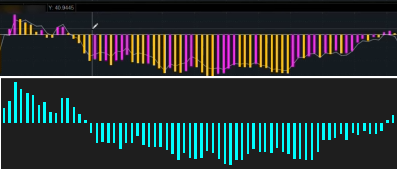It could be using the ETFs, or it could be using the Sector Indexes ( XLF versus \$SP500#40 ).

It may be using the change from the Open, or the change from the Prior Close.

And, It may be using a different weighting table than me. His info-tech is 27.9, but I used 29.2 from this weighting table, for example.

However, I've more or less deciphered it. I am decently certain that this is nonsense, and that it doesn't even use advance decline at all. It certainly does not use advance decline of individual sectors, that's for sure. Its based on the percentage change in the indexes -- basic average, and by weighting percentage.

Last edited:It could be using the ETFs, or it could be using the Sector Indexes ( XLF versus \$SP500#40 ).

It may be using the change from the Open, or the change from the Prior Close.

And, It may be using a different weighting table than me. His info-tech is 27.9, but I used 29.2 from this weighting table, for example.

However, I've more or less deciphered it. I am decently certain that this is nonsense, and that it doesn't even use advance decline at all. It certainly does not use advance decline of individual sectors, that's for sure. Its based on the percentage change in the indexes -- basic average, and by weighting percentage.

Oh, interesting. So something like this?

(pseudo code)

sectors = [XLK, XLV, XY, ...]

getSize(SectorETF) { percentChange * sectorWeight }
• get the sum of all sizes for each sector
• get the average i.e., divide the sum by the count of sectors
• multiply by some scaling quotient
• plot values as a histogram chart
• overlay \$ADSPD line to expose areas of divergenceIt could be using the ETFs, or it could be using the Sector Indexes ( XLF versus \$SP500#40 ).

It may be using the change from the Open, or the change from the Prior Close.

And, It may be using a different weighting table than me. His info-tech is 27.9, but I used 29.2 from this weighting table, for example.

However, I've more or less deciphered it. I am decently certain that this is nonsense, and that it doesn't even use advance decline at all. It certainly does not use advance decline of individual sectors, that's for sure. Its based on the percentage change in the indexes -- basic average, and by weighting percentage.

Could you share your code for the cyan histogram bars? I'd love to see and learn from your version.

•Mortimer
This is what I have so far. Not a bad start, but there are some issues I need to resolve.

https://tos.mx/id4OV5H

# # Sources:
# https://siblisresearch.com/data/us-sector-weightings/
# https://seekingalpha.com/etfs-and-funds/etf-tables/sectors
# debug
def transpose = .05;
def SectorCount = 11;
def NewDay = secondsFromTime(0930) < secondsFromTime(0930);
def InformationTechnology = 27.63; #XLK
def HealthCare = 13.25; #XLV
def ConsumerDiscretionary = 12.36; #XLY
def Financials = 11.39; #XLF
def Communications = 11.29; #XLC
def Industrials = 8.04; #XLI
def ConsumerStaples = 5.77; #XLP
def Energy = 2.75; #XLE
def RealEstate = 2.58; #XLRE
def Materials = 2.48; #XLB
def Utilities = 2.46; #XLU
def xlkWeight = InformationTechnology;
def xlvWeight = HealthCare;
def xlyWeight = ConsumerDiscretionary;
def xlfWeight = Financials;
def xlcWeight = Communications;
def xliWeight = Industrials;
def xlpWeight = ConsumerStaples;
def xleWeight = Energy;
def xlreWeight = RealEstate;
def xlbWeight = Materials;
def xluWeight = Utilities;
def xlk = close("XLK");
def xlv = close("XLV");
def xly = close("XLY");
def xlf = close("XLF");
def xlc = close("XLC");
def xli = close("XLI");
def xlp = close("XLP");
def xle = close("XLE");
def xlre = close("XLRE");
def xlb = close("XLB");
def xlu = close("XLU");
def xlkChangePercent = (xlk - xlk) / xlk;
def xlvChangePercent = (xlv - xlv) / xlv;
def xlyChangePercent = (xly - xly) / xly;
def xlfChangePercent = (xlf - xlf) / xlf;
def xlcChangePercent = (xlc - xlc) / xlc;
def xliChangePercent = (xli - xli) / xli;
def xlpChangePercent = (xlp - xlp) / xlp;
def xleChangePercent = (xle - xle) / xle;
def xlreChangePercent = (xlre - xlre) / xlre;
def xlbChangePercent = (xlb - xlb) / xlb;
def xluChangePercent = (xlu - xlu) / xlu;
def xlkSizing = xlkChangePercent * xlkWeight;
def xlvSizing = xlvChangePercent * xlvWeight;
def xlySizing = xlyChangePercent * xlyWeight;
def xlfSizing = xlfChangePercent * xlfWeight;
def xlcSizing = xlcChangePercent * xlcWeight;
def xliSizing = xliChangePercent * xliWeight;
def xlpSizing = xlpChangePercent * xlpWeight;
def xleSizing = xleChangePercent * xleWeight;
def xlreSizing = xlreChangePercent * xlreWeight;
def xlbSizing = xlbChangePercent * xlbWeight;
def xluSizing = xluChangePercent * xluWeight;
def combinedSizing = ((
xlkSizing +
xlvSizing +
xlySizing +
xlfSizing +
xlcSizing +
xliSizing +
xlpSizing +
xleSizing +
xlreSizing +
xlbSizing +
xluSizing
) / SectorCount);
def cumuCombinedSizing = if newday then combinedSizing else cumuCombinedSizing + combinedSizing;
# Display
AddLabel(yes, "InfoTech " + AsPercent(xlkSizing), if xlkSizing > 0 then color.GREEN else color.RED);
plot zeroLine = 0;
zeroLine.AssignValueColor(color.WHITE);
plot cumuSectorWeightAD = cumuCombinedSizing + transpose;

•Mortimer and desert_rat
This is what I have so far. Not a bad start, but there are some issues I need to resolve.

https://tos.mx/id4OV5H

# # Sources:
# https://siblisresearch.com/data/us-sector-weightings/
# https://seekingalpha.com/etfs-and-funds/etf-tables/sectors
# debug
def transpose = .05;
def SectorCount = 11;
def NewDay = secondsFromTime(0930) < secondsFromTime(0930);
def InformationTechnology = 27.63; #XLK
def HealthCare = 13.25; #XLV
def ConsumerDiscretionary = 12.36; #XLY
def Financials = 11.39; #XLF
def Communications = 11.29; #XLC
def Industrials = 8.04; #XLI
def ConsumerStaples = 5.77; #XLP
def Energy = 2.75; #XLE
def RealEstate = 2.58; #XLRE
def Materials = 2.48; #XLB
def Utilities = 2.46; #XLU
def xlkWeight = InformationTechnology;
def xlvWeight = HealthCare;
def xlyWeight = ConsumerDiscretionary;
def xlfWeight = Financials;
def xlcWeight = Communications;
def xliWeight = Industrials;
def xlpWeight = ConsumerStaples;
def xleWeight = Energy;
def xlreWeight = RealEstate;
def xlbWeight = Materials;
def xluWeight = Utilities;
def xlk = close("XLK");
def xlv = close("XLV");
def xly = close("XLY");
def xlf = close("XLF");
def xlc = close("XLC");
def xli = close("XLI");
def xlp = close("XLP");
def xle = close("XLE");
def xlre = close("XLRE");
def xlb = close("XLB");
def xlu = close("XLU");
def xlkChangePercent = (xlk - xlk) / xlk;
def xlvChangePercent = (xlv - xlv) / xlv;
def xlyChangePercent = (xly - xly) / xly;
def xlfChangePercent = (xlf - xlf) / xlf;
def xlcChangePercent = (xlc - xlc) / xlc;
def xliChangePercent = (xli - xli) / xli;
def xlpChangePercent = (xlp - xlp) / xlp;
def xleChangePercent = (xle - xle) / xle;
def xlreChangePercent = (xlre - xlre) / xlre;
def xlbChangePercent = (xlb - xlb) / xlb;
def xluChangePercent = (xlu - xlu) / xlu;
def xlkSizing = xlkChangePercent * xlkWeight;
def xlvSizing = xlvChangePercent * xlvWeight;
def xlySizing = xlyChangePercent * xlyWeight;
def xlfSizing = xlfChangePercent * xlfWeight;
def xlcSizing = xlcChangePercent * xlcWeight;
def xliSizing = xliChangePercent * xliWeight;
def xlpSizing = xlpChangePercent * xlpWeight;
def xleSizing = xleChangePercent * xleWeight;
def xlreSizing = xlreChangePercent * xlreWeight;
def xlbSizing = xlbChangePercent * xlbWeight;
def xluSizing = xluChangePercent * xluWeight;
def combinedSizing = ((
xlkSizing +
xlvSizing +
xlySizing +
xlfSizing +
xlcSizing +
xliSizing +
xlpSizing +
xleSizing +
xlreSizing +
xlbSizing +
xluSizing
) / SectorCount);
def cumuCombinedSizing = if newday then combinedSizing else cumuCombinedSizing + combinedSizing;
# Display
AddLabel(yes, "InfoTech " + AsPercent(xlkSizing), if xlkSizing > 0 then color.GREEN else color.RED);
plot zeroLine = 0;
zeroLine.AssignValueColor(color.WHITE);
plot cumuSectorWeightAD = cumuCombinedSizing + transpose;
They use the open to start the calculation. The line is for the 11 unweighted sectors and the histogram is for the weighted sectors.

•Mortimer and desert_ratIt could be using the ETFs, or it could be using the Sector Indexes ( XLF versus \$SP500#40 ).

It may be using the change from the Open, or the change from the Prior Close.

And, It may be using a different weighting table than me. His info-tech is 27.9, but I used 29.2 from this weighting table, for example.

However, I've more or less deciphered it. I am decently certain that this is nonsense, and that it doesn't even use advance decline at all. It certainly does not use advance decline of individual sectors, that's for sure. Its based on the percentage change in the indexes -- basic average, and by weighting percentage.
They use the change from the open. Their Info-tech weight is 29.3, pretty much the same as yours. They use the ETFs as I indicated in my first reply.

Last edited: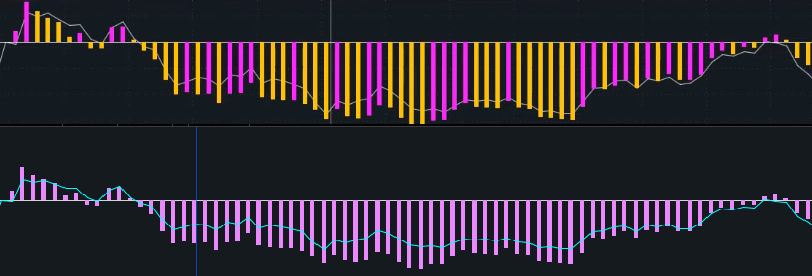Its the percentage change, from the close of the first bar, of all the sectors, multiplied by weight, added together, and brought to scale. Which is totally pointless, because SPX is already weighted properly to begin with; the percentage change of SPX itself already accomplishes this perfectly. This is just a bad measurement of the percent change in SPX with a bunch of ridiculous extra steps. To cap it off, all the final values are multiplied by five for no reason.

Here it is with SPX's percentage change from the close of its first bar (yellow):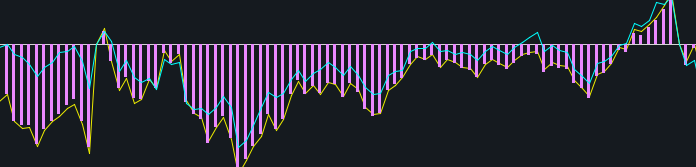Here is the closing price of the first bar of SPY (blue dash) :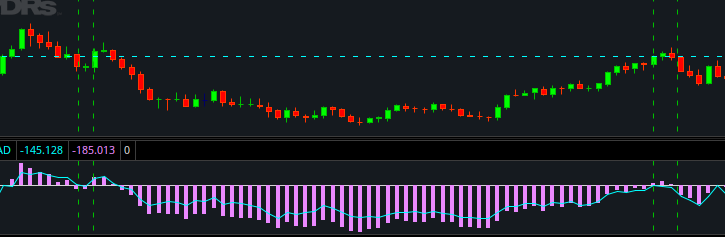The indicator will just cross the zero line when ever SPX (spy in this image) crosses above or below the close of its first bar...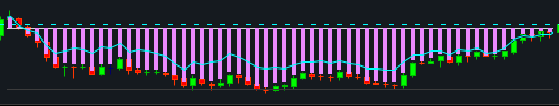Last edited:
Wow... so completely useless, and yet another marketing gimmick.

Thanks for the heads up @Joshua ... will not waste any more time on this nonsense.

I for one am still interested in this weighted advance decline idea. Anybody have any experience with this script?

I would still be interested.

Its the percentage change, from the close of the first bar, of all the sectors, multiplied by weight, added together, and brought to scale. Which is totally pointless, because SPX is already weighted properly to begin with; the percentage change of SPX itself already accomplishes this perfectly. This is just a bad measurement of the percent change in SPX with a bunch of ridiculous extra steps. To cap it off, all the final values are multiplied by five for no reason.

Here it is with SPX's percentage change from the close of its first bar (yellow):

Here is the closing price of the first bar of SPY (blue dash) :

The indicator will just cross the zero line when ever SPX (spy in this image) crosses above or below the close of its first bar...
If its not much to ask for, could it still be possible for you to share this script here or something similar? Thanks in advance

•Mortimer
It could be done (since they have at that site and want \$95 for their work).

I don't know what the sector tickers are through ThinkOrSwim, and I stole these from the trading view website, and I don't know the weightings, but it might go something like this:

Code:
``````declare lower;

def S5CONDD = close(symbol = "S5CONDD");  #  CONSUMER DISCRETIONARY
def S5CONSD = close(symbol = "S5CONSD");  #  CONSUMER STAPLES
def S5HLTHD = close(symbol = "S5HLTHD");  #  HEALTH CARE
def S5INDUD = close(symbol = "S5INDUD");  #  INDUSTRIALS
def S5INFTD = close(symbol = "S5INFTD");  #  INFORMATION TECHNOLOGY
def S5MATRD = close(symbol = "S5MATRD");  #  MATERIALS
def S5REASD = close(symbol = "S5REASD");  #  REAL ESTATE
def S5TELSD = close(symbol = "S5TELSD");  #  COMMUNICATION SERVICES
def S5UTILD = close(symbol = "S5UTILD");  #  UTILITIES
def SPFD    = close(symbol = "SPFD");     #  FINANCIALS
def SPND    = close(symbol = "SPND");     #  ENERGY

def S5CONDD_Weight = 1.0;
def S5CONSD_Weight = 1.0;
def S5HLTHD_Weight = 1.0;
def S5INDUD_Weight = 1.0;
def S5INFTD_Weight = 1.0;
def S5MATRD_Weight = 1.0;
def S5REASD_Weight = 1.0;
def S5TELSD_Weight = 1.0;
def S5UTILD_Weight = 1.0;
def SPFD_Weight = 1.0;
def SPND_Weight = 1.0;

AddLabel(yes, "S5CONDD  " + S5CONDD, color.black);
AddLabel(yes, "S5CONSD  " + S5CONSD, color.black);
AddLabel(yes, "S5HLTHD  " + S5HLTHD, color.black);
AddLabel(yes, "S5INDUD  " + S5INDUD, color.black);
AddLabel(yes, "S5INFTD  " + S5INFTD, color.black);
AddLabel(yes, "S5MATRD  " + S5MATRD, color.black);
AddLabel(yes, "S5REASD  " + S5REASD, color.black);
AddLabel(yes, "S5TELSD  " + S5TELSD, color.black);
AddLabel(yes, "S5UTILD  " + S5UTILD, color.black);
AddLabel(yes, "SPFD  "    + SPFD,    color.black);
AddLabel(yes, "SPND  "    + SPND,    color.black);

plot weighted_total = (S5CONDD * S5CONDD_weight) + (S5CONSD * S5CONSD_weight) + (S5HLTHD * S5HLTHD_weight) + (S5INDUD * S5INDUD_weight) + (S5INFTD * S5INFTD_weight) + (S5MATRD * S5MATRD_weight) + (S5REASD * S5REASD_weight) + (S5TELSD * S5TELSD_weight) + (S5UTILD * S5UTILD_weight) + (SPFD * SPFD_weight) + (SPND * SPND_weight);

plot unweighted_total = (S5CONDD + S5CONSD + S5HLTHD + S5INDUD + S5INFTD + S5MATRD + S5REASD + S5TELSD + S5UTILD + SPFD + SPND) / 11;``````

-mashume

Last edited:
•It could be done (since they have at that site and want \$95 for their work).

I don't know what the sector tickers are through ThinkOrSwim, and I stole these from the trading view website, and I don't know the weightings, but it might go something like this:

Code:
``````declare lower;

def S5CONDD = close(symbol = "S5CONDD");  #  CONSUMER DISCRETIONARY
def S5CONSD = close(symbol = "S5CONSD");  #  CONSUMER STAPLES
def S5HLTHD = close(symbol = "S5HLTHD");  #  HEALTH CARE
def S5INDUD = close(symbol = "S5INDUD");  #  INDUSTRIALS
def S5INFTD = close(symbol = "S5INFTD");  #  INFORMATION TECHNOLOGY
def S5MATRD = close(symbol = "S5MATRD");  #  MATERIALS
def S5REASD = close(symbol = "S5REASD");  #  REAL ESTATE
def S5TELSD = close(symbol = "S5TELSD");  #  COMMUNICATION SERVICES
def S5UTILD = close(symbol = "S5UTILD");  #  UTILITIES
def SPFD    = close(symbol = "SPFD");     #  FINANCIALS
def SPND    = close(symbol = "SPND");     #  ENERGYdef S5CONDD = close(symbol = "S5CONDD");  #  CONSUMER DISCRETIONARY

def S5CONDD_Weight = 1.0;
def S5CONSD_Weight = 1.0;
def S5HLTHD_Weight = 1.0;
def S5INDUD_Weight = 1.0;
def S5INFTD_Weight = 1.0;
def S5MATRD_Weight = 1.0;
def S5REASD_Weight = 1.0;
def S5TELSD_Weight = 1.0;
def S5UTILD_Weight = 1.0;
def SPFD_Weight = 1.0;
def SPND_Weight = 1.0;

AddLabel(yes, "S5CONDD  " + S5CONDD, color.black);
AddLabel(yes, "S5CONSD  " + S5CONSD, color.black);
AddLabel(yes, "S5HLTHD  " + S5HLTHD, color.black);
AddLabel(yes, "S5INDUD  " + S5INDUD, color.black);
AddLabel(yes, "S5INFTD  " + S5INFTD, color.black);
AddLabel(yes, "S5MATRD  " + S5MATRD, color.black);
AddLabel(yes, "S5REASD  " + S5REASD, color.black);
AddLabel(yes, "S5TELSD  " + S5TELSD, color.black);
AddLabel(yes, "S5UTILD  " + S5UTILD, color.black);
AddLabel(yes, "SPFD  "    + SPFD,    color.black);
AddLabel(yes, "SPND  "    + SPND,    color.black);

plot sumTotal = (S5CONDD * S5CONDD_weight) + (S5CONSD * S5CONSD_weight) + (S5HLTHD * S5HLTHD_weight) + (S5INDUD * S5INDUD_weight) + (S5INFTD * S5INFTD_weight) + (S5MATRD * S5MATRD_weight) + (S5REASD * S5REASD_weight) + (S5TELSD * S5TELSD_weight) + (S5UTILD * S5UTILD_weight) + (SPFD * SPFD_weight) + (SPND * SPND_weight);``````

-mashume
Is this supposed work ?nothing's showing up

•desert_rat

### Not the exact question you're looking for?

87k+ Posts
295 Online## The Market Trading Game Changer

Join 2,500+ subscribers inside the useThinkScript VIP Membership Club
• Exclusive indicators
• Proven strategies & setups
• Private Discord community
• Exclusive members-only content
• 1 full year of unlimited support

What is useThinkScript?

useThinkScript is the #1 community of stock market investors using indicators and other tools to power their trading strategies. Traders of all skill levels use our forums to learn about scripting and indicators, help each other, and discover new ways to gain an edge in the markets.

How do I get started?

We get it. Our forum can be intimidating, if not overwhelming. With thousands of topics, tens of thousands of posts, our community has created an incredibly deep knowledge base for stock traders. No one can ever exhaust every resource provided on our site.

If you are new, or just looking for guidance, here are some helpful links to get you started.

What are the benefits of VIP Membership?